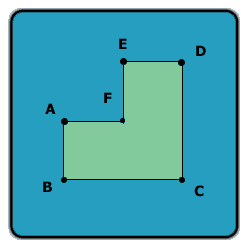All about flooble | fun stuff | Get a free chatterbox | Free JavaScript | Avatarsperplexus dot infoA different angle (Posted on 2003-10-10)Look at this shape:Assume AB = AF = FE = ED and BC = CD, and all the angles in the shape are 90 degrees.

Let A be the area of this shape (in cm^2), and P -- the perimeter of this shape (in cm).

If A - P is 60, what is the length of line AC?

 See The Solution Submitted by Lewis Rating: 1.9167 (12 votes)Comments: ( Back to comment list | You must be logged in to post comments.)A different angle| Comment 2 of 23 |this was easy as we have been doing triganometry at school these past weeks.
i got this because the preimeter is 8ab or 8af etc
and the area is (af x ba)+(ed x cd). if i put this in to a ratio it would equal area:perimeter 3:8. i then use the information to work out a-p=60.
so if 3-8 had to equal 60 i would have to times the area and perimeter -12. this gave me the equation -36+96=60 which is equal on each side.
i then used the phyphagourous (pardon my spelling) to find A to C. this worked out like
12² + 24² =720 when i squared rooted it ,it came to 26.828.
thank you and now i can be a student
 Posted by Callum on 2003-10-10 08:01:31Please log in:

 Search: Search body:
Forums (5)